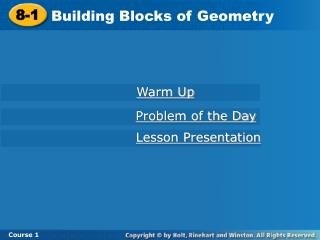DownloadDownload Presentation8-1

# 8-1

Download Presentation## 8-1

- - - - - - - - - - - - - - - - - - - - - - - - - - - E N D - - - - - - - - - - - - - - - - - - - - - - - - - - -
##### Presentation Transcript

1. 8-1 Building Blocks of Geometry Course 1 Warm Up Problem of the Day Lesson Presentation

2. Today you will begin a unit on Geometry.

3. OBJECTIVE FOR TODAY Learn to describe figures by using the terms of geometry.

4. Vocabulary point line plane line segment ray

5. A point is an exact location. P point P, P A point is named by a capital letter. A lineis a straight path that line AB, AB, extends without end in A B line BA, BA opposite directions. A line is named by two points on the line. M A plane is a flat surface that plane LMN, extends without end in all plane MLN, directions. plane NLM A plane is named by three points on the plane that are not on the same line. L N The building blocks of geometry are points, lines, and planes.

6. Q P R M N PR and NR You can also write RP and RN. Additional Example 1: Identifying Points, Lines, and Planes Use the diagram to name the geometric figure. A. three points M, N, and P Five points are labeled: points M, N, P, Q, and R. B. two lines

7. Point R is a point on PR and NR. Q P R M N Additional Example 1: Identifying Points, Lines, and Planes Use the diagram to name the geometric figure. C. a point shared by two lines point R D. a plane plane QRM Use any three points in the plane that are not on the same line. Write the three points in any order.

8. V U W T S UW and SW You can also write WU and WS. Check It Out: Example 1 Use the diagram to name the geometric figure. A. three points S, T, and U Five points are labeled: points S, T, U, V, and W. B. two lines

9. Point W is a point on WS and WU. V U W T S Check It Out: Example 1 Use the diagram to name the geometric figure. C. a point shared by two lines point W D. a plane plane VUT Use any three points in the plane that are not on the same line. Write the three points in any order.

10. A line segment is line segment XY, XY, made of two endpoints Y line segment YX, YX and all the points between X the endpoints. A line segment is named by its endpoints. A rayhas one endpoint. ray JK, JK From the endpoint, the ray J K extends without end in one direction only. A ray is named by its endpoint first followed by another point on the ray.

11. B A C AB, BC, and AC You can also write BA, CB, and CA. AB, BC, and AC You can also write BA, CB, and CA. Additional Example 2: Identifying Line Segments and Rays Use the diagram to give a possible name to the figure. A. three different line segments B. three ways to name the line

12. B AB, AC, BC, CB, CA, and BA A C AC Additional Example 2: Identifying Line Segments and Rays Use the diagram to give a possible name to the figure. C. six rays D. another name for ray AB A is still the endpoint. C is another point on the ray.

13. D E F DE, EF, and DF You can also write ED, FE, and FD. DE, EF, and DF You can also write ED, FE, and FD. Check It Out: Example 2 Use the diagram to give a possible name to the figure. A. three different line segments B. three ways to name the line

14. D DE, EF, DF, FE, FD, and ED E F DF Check It Out: Example 2 Use the diagram to give a possible name to the figure. C. six rays D. another name for ray DE D is still the endpoint. F is another point on the ray.

15. Possible answer: AD, CE Lesson Quiz Use the diagram to name each geometric figure. 1. three points 2. two lines 3. a point shared by a line and a ray 4. a plane Possible answer: A, B, C Possible answer: F Possible answer: plane AEC

16. What we learned today! Learned to describe figures by using the terms of geometry.